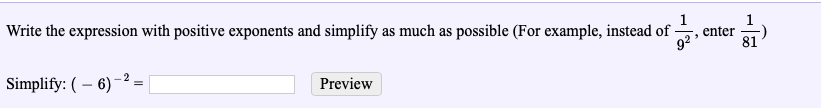# Write the expression with positive exponents and simplify as much as possible (For example, instead of -2, enter ^-)81Simplify: (- 6)Preview

Question
100 viewshelp_outlineImage TranscriptioncloseWrite the expression with positive exponents and simplify as much as possible (For example, instead of -2, enter ^-) 81 Simplify: (- 6) Preview fullscreen
check_circle

Step 1

Firstly, we use the definition of a  negative exponent , which states that x^(-n)=1/x^n.

Step 2

Then, we use the fact that (-a)^n =a^n if n...

### Want to see the full answer?

See Solution

#### Want to see this answer and more?

Solutions are written by subject experts who are available 24/7. Questions are typically answered within 1 hour.*

See Solution
*Response times may vary by subject and question.
Tagged in

### Exponents